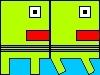#### Howdy, Stranger!

We are about to switch to a new forum software. Until then we have removed the registration on this forum.

# Character Physics

edited December 2017

I've recently been working on a game. I've ran into many problems making the game but thanks to to great people here I've been able to get thorugh it. I have another problem. Currently the physics with the character isn't working the best (I'm using the Box2D library). To make the hit box follow the sprite I had to add When I move the character along the x coridnate the gravity doesn't seem to effect them as much. Also if the character is moved it seems to bounce along a platform, the jump doesn't work (Line: 108, 141) and finally I can't get the bullets to follow the player (I don't know what variable to use) (Line:296,306). I would greatly appricate any assistance that could be offered as I feel I'm in the finishing stretch of the game.

``````//Minim

//import ddf.minim.analysis.*;
//import ddf.minim.*;
//Minim minim;
//AudioPlayer Tune;

//Sprites
PImage sprite1;//, sprite2;

//Box2D
import shiffman.box2d.*;
import org.jbox2d.collision.shapes.*;
import org.jbox2d.common.*;
import org.jbox2d.dynamics.*;
// A list for all of our rectangles
ArrayList<Box> boxes;
Box2DProcessing box2d;

//Variables
float x=0.0;
float y=0.0;
float frame=0;
int direction=0;
int fchange=0;
int jump=0;

float left=0;
float right=0;

//Controls
int w = 0;
int a = 0;
int s = 0;
int d = 0;
int q = 0;

Box h;
Box i;

int l = 0;

void setup()
{
//Base Setup
size(1280, 720, P2D);
frameRate(60);
smooth();

//minim = new Minim(this);
//Tune.loop();

textureMode(NORMAL);
blendMode(BLEND);
noStroke();

// Initialize and create the Box2D world
box2d = new Box2DProcessing(this);

box2d.createWorld();

// Turn on collision listening!
box2d.listenForCollisions();

// Create ArrayLists
boxes = new ArrayList<Box>();

//Top Platform
Box p = new Box(640, 325, 300, 30, 1, false);

//Middle Platform Left
Box b = new Box(150, 515, 300, 30, 1, false);

//Middle Platform Middle
Box c = new Box(640, 515, 100, 30, 1, false);

//Middle Platform Right
Box e = new Box(1130, 515, 300, 30, 1, false);

//Middle Colum
Box f = new Box(640, 420, 30, 160, 1, false);

//Bottom Platform
Box g = new Box(640, 705, 1280, 30, 1, false);

//Player 1
h = new Box(x+25, y+37.5, 50, 75, 0, true);
h.body.setGravityScale(9.8f);
if (jump==1)
{
h.body.setAngularVelocity(1);
h.body.setLinearVelocity(new Vec2(0, -10));
jump=0;
}
}
void draw()
{
background(0, 200, 255);

//Sun
fill(255, 255, 0);
ellipse(100, 100, 100, 100);

// We must always step through time!
box2d.step();
// Display all the boxes
for (Box b : boxes)
{
b.display();
}

//Player
left = frame/2;
right = (frame+1)/2;

if (direction==1)
{
float temp = left;
left = right;
right = temp;
}

//Controls
if (w==1)
{
jump = 1;
y=y-10;
}

if ((a==1)&(x!=0))
{
direction = 0;
x-=100;
h.body.setAngularVelocity(1);
h.body.setLinearVelocity(new Vec2(-100, 0));
if (fchange == 10)
{
frame++;
if (frame==2) frame=0;
fchange=fchange-10;
}

fchange++;
}

if ((d==1)&(x!=1280-50))
{
direction=1;
x+=100;
h.body.setAngularVelocity(1);
h.body.setLinearVelocity(new Vec2(100, 0));
if (fchange == 10)
{
frame++;
if (frame==2) frame=0;
fchange=fchange-10;
}
fchange++;
}

if (s==1)
{
h.body.setGravityScale(100.8f);
y=y+10;
}
}

// A rectangular box
class Box
{
// Instead of any of the usual variables,
// we will store a reference to a Box2D Body
Body body;
Vec2 pos;
float w, h;
boolean player1;
Box(float x, float y, float w_, float h_, int type, boolean player1)
{
this.player1 = player1;
w = w_;
h = h_;
// Build Body
BodyDef bd = new BodyDef();
if (type==0)
{
bd.type = BodyType.DYNAMIC; // Was DYNAMIC
} else
{
bd.type = BodyType.STATIC;
}
bd.position.set(box2d.coordPixelsToWorld(x, y));
body = box2d.createBody(bd);
// Define a polygon (this is what we use for a rectangle)
PolygonShape sd = new PolygonShape();
// Box2D considers the width and height of a
// rectangle to be the distance from the
// center to the edge (so half of what we
// normally think of as width or height.)
float box2dW = box2d.scalarPixelsToWorld(w/2);
float box2dH = box2d.scalarPixelsToWorld(h/2);
sd.setAsBox(box2dW, box2dH);

// Define a fixture
FixtureDef fd = new FixtureDef();
fd.shape = sd;
// Parameters that affect physics
fd.density = 1;
fd.friction = 100;
fd.restitution = 0;
// Attach Fixture to Body
body.createFixture(fd);
}
void display()
{
// We need the Body’s location and angle
pos = box2d.getBodyPixelCoord(body);
if(!player1)
{
pushMatrix();
translate(pos.x, pos.y);
fill(255, 0, 0);
stroke(0);
rectMode(CENTER);
rect(0, 0, w, h);
popMatrix();

}

if(player1)
{
pushMatrix();
translate(pos.x-25, pos.y-37.5);
beginShape();
noStroke();
vertex(0, 0, right, 0);
vertex(50, 0, left, 0);
vertex(50, 75, left, 1);
vertex(0, 75, right, 1);
endShape();
popMatrix();

}
}
} // End of the Box Class description

void keyPressed()
{
if (key=='w')
{
w = 1;
}

if (key=='d')
{
d = 1;
/*
Vec2 a = box2d.coordPixelsToWorld(h.pos.x+x, (h.pos.y));
h.body.setTransform( a, 0.0f);
*/

}

if (key=='a')
{
a = 1;
/*
Vec2 a = box2d.coordPixelsToWorld(h.pos.x+x, (h.pos.y));
h.body.setTransform( a, 0.0f);
*/
}

if (key=='s')
{
s = 1;
}

if ((key=='q')&&(direction==1))
{
i = new Box(x+55, y+35, 10, 10, 0, false);
i.body.setGravityScale(0f);
i.body.setAngularVelocity(1);
i.body.setLinearVelocity(new Vec2(1280, 0));
}
if ((key=='q')&&(direction==0))
{
i = new Box(x-5, y+35, 10, 10, 0, false);
i.body.setGravityScale(0f);
i.body.setAngularVelocity(1);
i.body.setLinearVelocity(new Vec2(-1280, 0));
}
}

void keyReleased()
{
if (key=='w')
{
w = 0;
}

if (key=='d')
{
d = 0;
}

if (key=='a')
{
a = 0;
}

if (key=='s')
{
s = 0;
}
}
``````